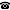# 2014 seminar talk: Topological dynamics of unordered Ramsey structures

Talk held by Moritz Müller (KGRC) at the KGRC seminar on 2014-05-15.

### Abstract

We study connections between Ramsey properties of Fraïssé classes $\mathcal{K}$ and the universal minimal flow $M(G_\mathcal{K})$ of the automorphism group $G_\mathcal{K}$ of their Fraïssé limits. For example, extending a result of Kechris, Pestov and Todorcevic, we show that if the class $\mathcal{K}$ has finite Ramsey degree for embeddings, then this degree equals the size of $M(G_\mathcal{K})$.

Kurt Gödel Research Center for Mathematical Logic. Währinger Straße 25, 1090 Wien, Austria.+43-1-4277-50501. Last updated: 2010-12-16, 04:37.